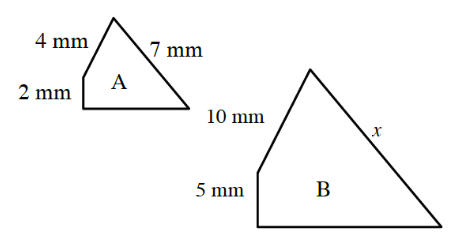### Home > GB8I > Chapter 7 Unit 8 > Lesson INT1: 7.1.1 > Problem7-8

7-8.

Jay enlarged figure $A$ so that it was similar to figure $B$. His diagram is shown at right. Homework Help ✎

1. What is the scale factor?

Read Math Notes box in Lesson 7.1.1 to determine how to write a scale factor.
Remember a scale factor is a ratio (fraction).

2. What is the value of $x$? How do you know?

Which side of figure A corresponds to side $x$ in figure $B$?
Multiply that side length by the scale factor you found in part (a).